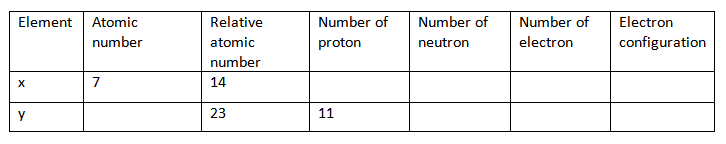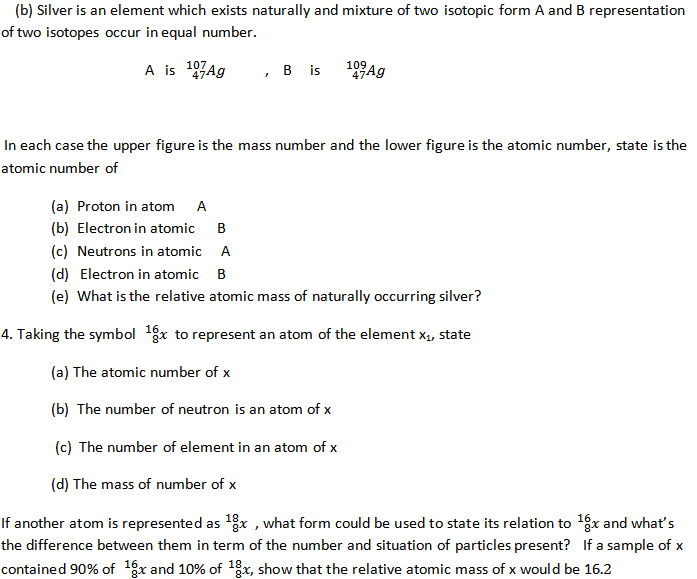CHEMISTRY O LEVEL(FORM TWO) – ATOMIC STRUCTURE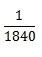Written by

ecolebooks.com

ATOMIC STRUCTURE
ATOM: Is the smallest particle of an element that can take part in a chemical reaction.
Atoms as the smallest particles have ability to exist on its own. Dalton was the first person to use the word ATOM.
DALTON ATOMIC THEORY
1. All matter are made up of tiny particles called ATOM.
2. Atoms can neither be created nor destroyed.
3. Atoms of a given element are identical. They have the same atomic mass and similar chemical properties.
4. Atoms of one elements can combine with atoms of other elements to form molecules.
5. Atoms of given element are different from those of other elements.
MODIFICATION OF DALTON ATOMIC THEORY

1. Atoms are made up of smaller particles called electrons, protons and neutrons.

2. Atom can be created or destroyed or split up by nuclear reaction/ nuclear fission.

3. Some element have atom of more than one type. They are called Isotopes.

4. Atoms of different elements combine together to form complex compound.

SUB ATOMIC PARTICLES
• Atom are made up of 3 particles these are;
– PROTON
– ELECTRON
– NEUTRON
• All atoms of an element have both 3 particles except hydrogen which has no Neutron.
A: THE ELECTRON
• This is a negatively charged particle (-ve)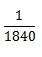• It’s symbol is ‘e
• It rotates around the nucleus in a particular patten called shell or energy level
B: THE PROTON
• This is a positively charged particles (+ve)
• It has mass approximately the same as that of hydrogen atom ie atomic mass
• It is symbol is (p) or +11P
• It is found in the nucleus of an atom
C: THE NEUTRON
• This is a neutral particle or is a particle which has .no charge
• The mass is the same as that of protons ie atomic mass unit
• It symbol is (n)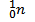• It is found in the nucleus of an atom
• summary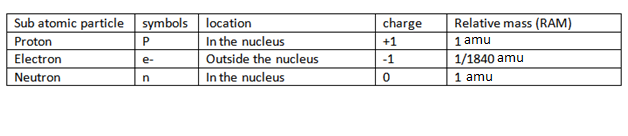• ATOMIC STRUCTURE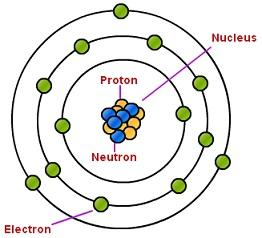Although an atom contain charge particles (protons) and electron is natural because the number of protons (+Ve) are equal to the number of electron (-Ve)
THE ARRANGEMENT OF ELECTRONS IN ATOM
ELECTRONIC CONFIGURATIONS
Electronic configuration: Is the distribution of electrons in various shell of an atom.
The maximum number of electrons held within each energy level. It can be determined by the formula 2n2
Where n is the position of energy level from the nucleus
• The first shell from the nucleus of an atom have ability of carrying only 2 electron. (2×12 ) = 2 electrons
• The second shell from the nucleus of an atom has ability of carrying only 8 electrons. ( 2×22) = 8 electrons
• The third shell from the nucleus of an atom have ability of carrying only 8 electronic. (2×32) =18 electrons
• The forth shell from the nucleus carry a maximum of 18 electrons. (2×42) = 32 electrons
NOTE; But the third energy level is stable with 8 electrons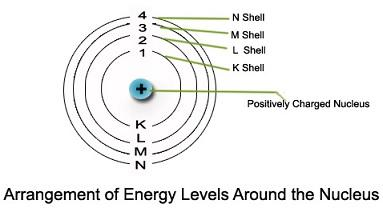Example of electron diagrams of an atom;

1. Hydrogen, 1=1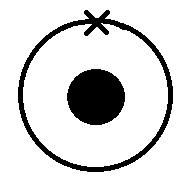2. Aluminium, 13=2:8:3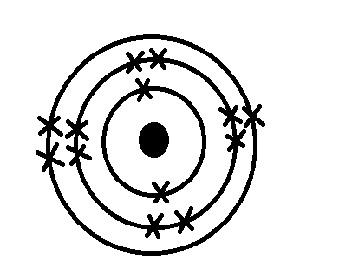3. Chlorine, 17 =2:8:7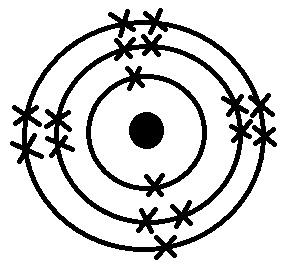4. Oxygen, 8= 2:6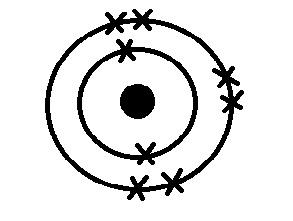ELECTRONS CONFIGURATIONS OF FIRST ELEMENT
 Element Symbol Number of neutron Atomic number/Proton and Electron Atomic Mass Electronic configuration (KLMN) Number of shell Hydrogen H 0 1 1 1 1 Helium He 2 2 4 2 1 Lithium Li 4 3 7 2:1 2 Beryllium Be 5 4 9 2:2 2 Boron B 6 5 11 2:3 2 Carbon C 6 6 12 2:4 2 Nitrogen N 7 7 14 2:5 2 Oxygen O 8 8 16 2:6 2 Florine F 10 9 19 2:7 2 Neon Ne 10 10 20 2:8 2 Sodium Na 12 11 23 2:8:1 3 Magnesium Mg 12 12 24 2:8:2 3 Aluminum Al 14 13 27 2:8:3 3 Silicon Si 14 14 28 2:8:4 3 Phosphorus P 16 15 31 2:8:5 3 Sulphur S 16 16 32 2:8:6 3 Chlorine Cl 18 17 35 2:8:7 3 Argon Ar 18 18 36 2:8:8 3 Potassium K 19 19 38 2:8:8:1 4 Calcium Ca 20 20 40 2:8:8:2 4
NOTE
1. In the above the element are arranged according to the increase in atomic number
2. The number of proton = number of electron = Atomic number
3. The mass number (A) is the sum of proton (P) and neutron (N): (A = P+N)
ATOMIC NUMBER, MASS NUMBER AND ISOTOPES
ATOMIC NUMBER (Z)
Is the number of proton in the nucleus of an atom which is equal to the number of electron in the shell.
• It’s official symbol is (Z).
• Atomic number is written on left hand side below the symbol of an element.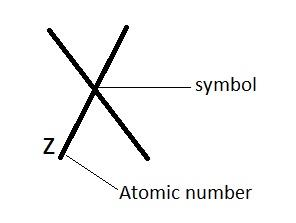2. MASS NUMBER / ATOMIC MASS (A)
• It is written on left hand side above the symbol of an element.
E.g;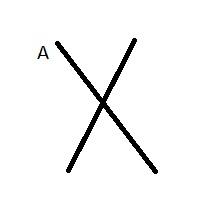.E.g. Carbon atomic mass is 12 written as 12C chlorine atomic mass is written as 35Cl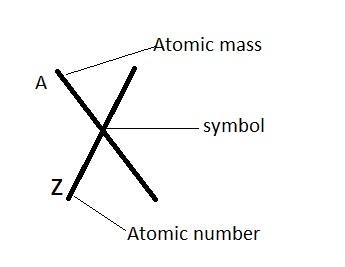e.g.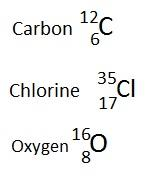Therefore Atomic mass = Proton (atomic number)
Example; Atom R has mass number of 40 and an atomic number of 20. What is it’s neutron number , and what is the number of electrons in an atom R ?

Solution ; mass number = 40
atomic number = 20

(a) Neutron number = mass number − atomic number

= 40 − 20
Neutron number = 20

(b) Number of electrons = number of protons = atomic number = 20

ISOTOPES

These are atoms of the same element which have the same atomic number but they differ in mass number.
• Isotopes have the same proton electron and atomic number.
• They have same chemical properties but have slight different physical properties.
• Isotopes has different mass number because they have different number of neutrons.
• Example of element which have the isotopes;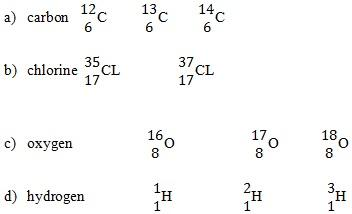NOTE:
RELATIVE ATOMIC MASS (RAM)
• The relative atomic mass of an element is the mass of an atom of carbon twelve(12) Isotopes .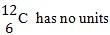• If an element has several Isotopes its relative atomic mass will be the mass of Isotopes on calculation. The average mass of the proportion (abundance) of each Isotope in the sample of element must be known.
• This is calculated by working out the relative abundance of each isotope
CALCULATING RELATIVE ATOMIC NUMBER

Relative atomic mass (RAM) =( Relative abundance × Atomic mass ) + ( Relative abundance × Atomic mass )
100

Example:

1. A sample of chlorine gas contains 75% and 25% of the Isotopes with it’s relative abundance of 35 and 25 respectively. What is the relative atomic mass (R.A.M) of chlorine?
RAM =( Relative abundance × Atomic mass ) + ( Relative abundance × Atomic mass)
100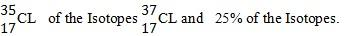To get the answer multiply the mass number of each Isotopes with the abundance.
Solution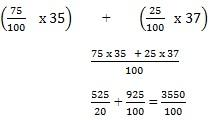R.A.M of chlorine = 35.5
• The relative atomic mass of chlorine is 35. 5. The word symbol of relative atomic mass is (RAM).

2. A sample of chlorine is a mixture of two Isotopes in the ratio of 3:1. What is the relative atomic mass of chlorine atom?
SolutionR.A.M of chlorine = 35.5

3. A sample of Oxygen is mixture of 3 Isotopes in the ratio of 3:2:1. What is relative atomic mass (R.A.M).
Solution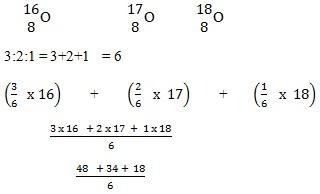R.A.M of Oxygen = 16.6
REVIEW QUESTION

1. Define the following

(i) Atomic

(ii) Electronic configuration

(iii) Atomic number

(iv) Mass number

(V) Isotope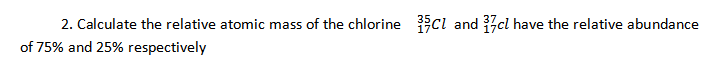3. Copy and complete the following table of atomic / electron structure of same element

(a).